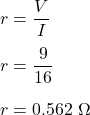## You short-circuit a 9 volt battery by connecting a short wire from one end of the battery to the other end. If the current in the short circ

Question

You short-circuit a 9 volt battery by connecting a short wire from one end of the battery to the other end. If the current in the short circuit is measured to be 16 amperes, what is the internal resistance of the battery?

in progress 0
6 months 2021-07-29T00:23:54+00:00 1 Answers 5 views 0

## Answers ( )

The internal resistance of the battery is 0.562 ohms.

Explanation:

Given that,

Voltage of battery, V = 9 volts

Current in the short circuit, I = 16 A

• In case of short circuit, the external resistance of the circuit will be equal to 0.

So, we can say that, the internal resistance of the battery is given by :So, the internal resistance of the battery is 0.562 ohms.